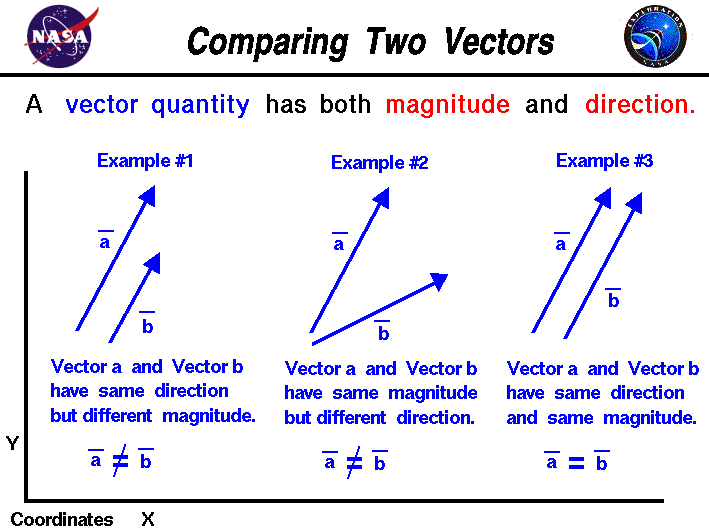+ Text Only Site
+ Non-Flash Version
+ Contact GlennMath and science were invented by humans to describe and understand the world around us. We observe that there are some quantities and processes in our world that depend on the direction in which they occur, and there are some quantities that do not depend on direction. Mathematicians and scientists call a quantity which depends on direction a vector quantity. A quantity which does not depend on direction is called a scalar quantity. A vector quantity has two characteristics, a magnitude and a direction. When comparing two vector quantities of the same type, you have to compare both the magnitude and the direction. On this slide we show three examples in which two vectors are being compared. Vectors are usually denoted on figures by an arrow. The length of the arrow indicates the magnitude and the tip of the arrow indicates the direction. The vector is labeled with an alphabetical letter with a line over the top to distinguish it from a scalar. Our web print fonts don't allow for this notation, so we will use a bold letter for a vector. We will be comparing two vectors, a and b. They could be forces, or velocities, or accelerations; it doesn't really matter. Example #1: We have two vectors with the same direction, but the magnitudes (or length of the vectors) are different. Vector a does not equal vector b in this example. This example seems pretty simple, because the same rule applies for scalars; if the magnitude is different, the quantities are not equal. An object weighing 50 pounds is not equal to an object weighing 25 pounds. Example #2: This example is a little more complex. In this case, we have two vectors with equal magnitude, but the directions are different. Vector a does not equal vector b in this example. If the vector was a velocity, this tells us that a car traveling 45 mph to the northeast will end up in a different place than another car also traveling 45 mph due east. In one hour, they will both move 45 miles, but the locations will be different. In two hours, they will be even farther apart. Example #3: In this example, we have two vectors with equal length and equal direction. Vector is equal to vector b. For two vectors to be equal, they must have both the magnitude and the directions equal. Guided Tours Activities: Related Sites: Rocket Index Rocket Home Beginner's Guide Home+ Inspector General Hotline + Equal Employment Opportunity Data Posted Pursuant to the No Fear Act + Budgets, Strategic Plans and Accountability Reports + Freedom of Information Act + The President's Management Agenda + NASA Privacy Statement, Disclaimer, and Accessibility CertificationEditor: Tom Benson NASA Official: Tom Benson Last Updated: May 13 2021 + Contact Glenn